Latest Banking jobs   »   Quantitative Aptitude Quiz For SBI/IBPS PO...

# Quantitative Aptitude Quiz For SBI/IBPS PO Mains 2021- 26th December

Directions (1-5): Pie chart shows the % distribution of profit of a man after selling 5 items. Graph shows his profit% earned and discount% given by him on each items.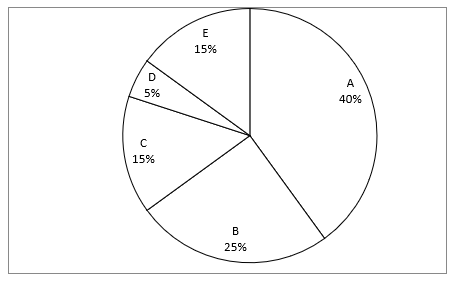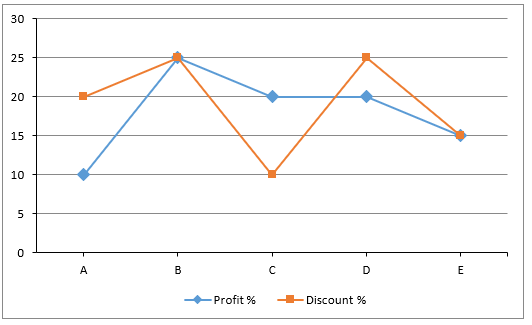NOTE:- All profit are calculated on single article of each item.

Q1. If total profit earned by him on selling all item is Rs. 10000, then what is difference between MRP of item A and item D?
(a) 47000
(b) 51000
(c) 13000
(d) 11500
(e) None of these

Q2. SP of item B is what % more or less than MP of item C.
(a) 15
(b) 20
(c) 25
(d) 30
(e) Can’t be determined

Q3. What is profit% earned by men on item B and D together?
(a) 24%
(b) 30%
(c) 23.07%
(d) 100%
(e) Can’t be determined

Q4. What is the ratio of MP of item C to SP of item E?
(a) 2 : 1
(b) 21 : 13
(c) 13 : 15
(d) 20 : 23
(e) None of these

Q5. If the above data is prepared by his son and he calculated his total profit of Rs. 10000. But in evening he revealed to his son, that actually he marked up the price of item C by twice and he himself got a discount of 40% on original price of item C. Find his actual profit amount on item C?
(a) Rs. 3000
(b) Rs. 4500
(c) Rs. 6000
(d) Rs. 7500
(e) Rs. 9000

Directions (6-7): A hollow cylinder, opened from both ends is having inner radius 7 cm, height 14 cm and thickness 1 cm.

Q6. If this cylinder is to be melted to form identical spheres of radius 2.1 cm. How many spheres could be formed approximately?
(a) 28
(b) 17
(c) 21
(d) 29
(e) 25

Q7. If this hollow cylinder is melted and casted into a solid(not hollow) cube. Find the side[approximate] of cube formed?
(a) 3.26
(b) 4.18
(c) 2.75
(d) 8.70
(e) 10.12

Directions (8-10): In the given questions, two quantities are given, one as ‘Quantity I’ and another as ‘Quantity II’. You have to determine relationship between two quantities and choose the appropriate option:

Q8. A man invested in the ratio of 8 : 11 in two schemes ‘A’ & B. Scheme ‘A’ offered compound interest, while scheme ‘B’ offered simple interest.
Quantity I – Amount invested in scheme ‘B’.
If rate of interest offered in scheme ‘A’ & ‘B’ was 20% p.a. and 16% p.a. respectively and the man got Rs. 3520 as total interest from both schemes after two years.
Quantity II – Amount invested by man in scheme ‘A’.
If rate of interest offered in scheme ‘A’ & ‘B’ was 10% p.a. and 20% p.a. respectively and man got Rs. 1870 more as interest from scheme ‘B’ as compared to A after two years.
(a) Quantity I > Quantity II
(b) Quantity I < Quantity II
(c) Quantity I ≥ Quantity II
(d) Quantity I ≤ Quantity II
(e) Quantity I = Quantity II or no relation

Q9. A bag contains 9 yellow balls, Y green balls and 7 red balls, if one ball is drawn at random from bag probability of it being green is 5/21 .
Quantity I – The probability that at least one of the fruits is apple when, two fruits are taken out from bucket P without replacement.
Bucket ‘P’ contains 2Y apple, 3(Y – 2) banana & 1.5(Y – 1) orange.
Quantity II – The probability that both of the dice are of same color, when two dice are taken out from bucket Q without replacement.
Bag ‘Q’ contains 4 red dice, 3.5(Y – 3) green dice and 2Y yellow dice.
(a) Quantity I > Quantity II
(b) Quantity I < Quantity II
(c) Quantity I ≥ Quantity II
(d) Quantity I ≤ Quantity II
(e) Quantity I = Quantity II or no relation

Q10. Two vessel A & B contains mixture of milk & water in the quantity of (X + 54) l and (X + 84) l respectively. The ratio of milk & water in vessel A and vessel B is 3 : 2 and 2 : 1 respectively.
Quantity I – Quantity of milk in vessel B.
If 60% mixture from vessel A and 66 ⅔% mixture from vessel B taken out, then the remaining mixture in both vessels is equal.
Quantity II – 144 l
(a) Quantity I > Quantity II
(b) Quantity I < Quantity II
(c) Quantity I ≥ Quantity II
(d) Quantity I ≤ Quantity II
(e) Quantity I = Quantity II or no relation

Solutions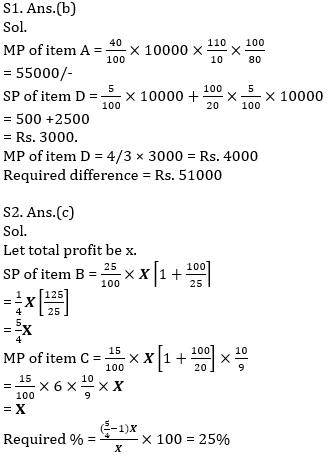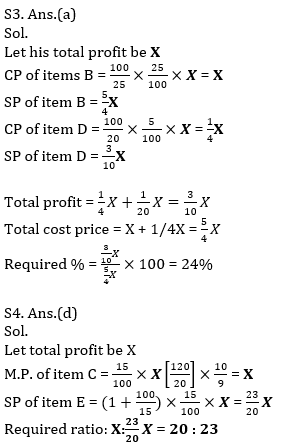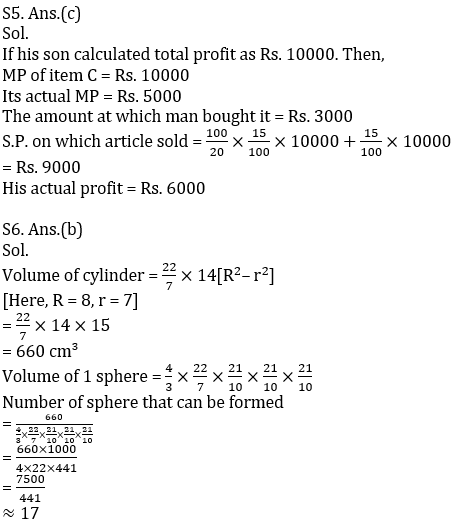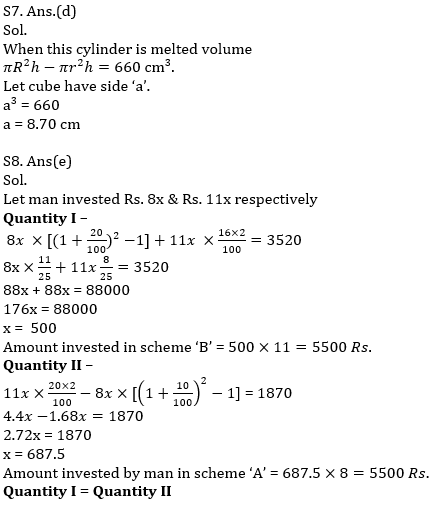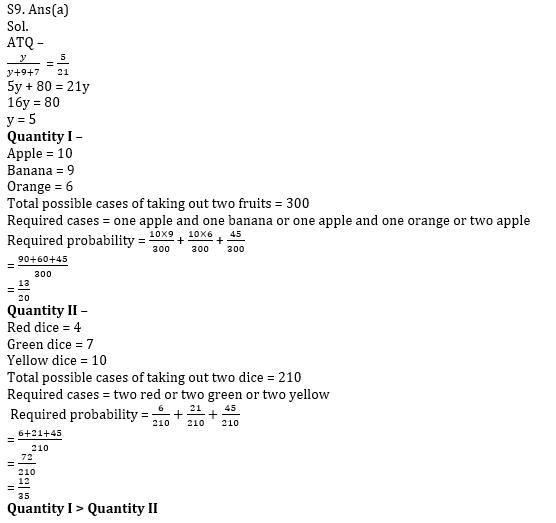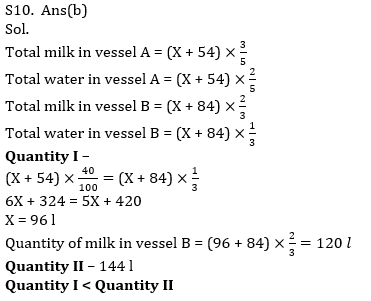#### Congratulations!Incorrect details? Fill the form again here

•Quantitative Aptitude Quiz For IBPS RRB ...
•Quantitative Aptitude Quiz For IBPS Cler...
•Quantitative Aptitude Quiz For IDBI AM/E...
•Quantitative Aptitude Quiz For IDBI AM/E...
•Quantitative Aptitude Quiz For SBI Clerk...
•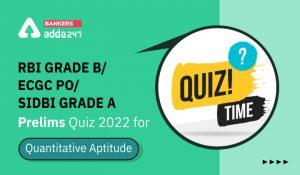Quantitative Aptitude Quiz For RBI Grade...